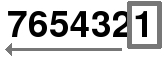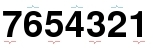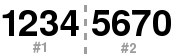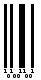:

# EAN 8 BARCODE

## Format of the EAN 8

Length Type checksum
8 digits numeric yes

The EAN 8 is composed by 7 data digits + 1 checksum digit.

The EAN 8 is derived from EAN 13. It is based on the same tables as EAN 13 and has the same checksum calculation.

## Calculating the EAN 8 checksum

Calculating the EAN 8 checksum digit is very simple :

• The digit are used from right to left• Calculate the sum of the numbers of odd and even positions• Odd : 1 + 3 + 5 + 7 = 16
• Even : 2 + 4 + 6 = 12

### EAN 8 checksum formula :

( 10 - [ (3 * Odd + Even) modulo 10 ] ) modulo 10

Thus, the example :

• (10 - [ (3 * 16 + 12) module 10 ] ) modulo 10
• (10 - [ (48 + 12) module 10 ] ) modulo 10
• (10 - [ (60) module 10 ] ) modulo 10
• (10 - [ 0 ] ) modulo 10
• (10 - 0) modulo 10
• 10 modulo 10
• 0

So, the checksum of 7654321 is 0

### EAN 8 checksum generator :

EAN 8 message : Checksum :

## Binary sequence of EAN 8 barcode

#### Start

A start sequence starts the barcode : 101

The 8 digits will be separated into 2 parts, namely the left part from the 1st to the 4th digit and the right part from the 5th to the 8th digit#### Part #1

The first part is encoded according to the following table :

digitsequence
00001101
10011001
20010011
30111101
40100011
50110001
60101111
70111011
80110111
90001011

Part #1 will be 0011001 0010011 0111101 0100011

#### Intermediate

An intermediate sequence separate the 2 parts : 01010

#### Part #2

The second part is encoded according to the following table :

digitséquence
01110010
11100110
21101100
31000010
41011100
51001110
61010000
71000100
81001000
91110100

Part #2 will be 1001110 1010000 1000100 1110010

#### Stop

A stop sequence ends the barcode : 101

### Summary of the sequence of the EAN 8

• start : 101
• part #1
• center : 01010
• part #2
• stop : 101

## Graphical representation of the EAN 8 barcode

Each element of the binary sequence is represented by a space of equal width, it is a "1" or "0". The "1" are represented by spaces filled and "0" with empty spaces.## Online EAN 8 barcode generator

Please fill in the code :